• 在这个项目中，我们要处理使用EM算法训练GMM-HMM的孤立单词数据。 测试阶段也考虑使用维特比算法。 结果表明，通过Matlab 编程获得的性能与HTK 的性能相似。 在运行这些程序之前，请先准备好训练和测试数据。 ...matlab
• hmm模型matlab代码HMM-GMM 这是我个人实现的隐马尔可夫模型和高斯混合模型，这是统计机器学习中的两个经典生成模型。 HMM是在无监督的情况下进行训练的，代码实现了前向后退算法，以在给出部分/全部观测值的任何时间...
• hmm模型matlab代码Mattia的ML工具 高斯混合模型，隐马尔可夫模型和相关算法的另一个Matlab实现。 为学习而构建，用于下面引用的我的HRI'18论文。 该代码使用了Tom Minka的两个库： 快速安装： 光速： 详细： 高斯...

GMM-HMM语音识别

为了对GMM-HMM在语音识别上的应用有个宏观认识，花了些时间读了下HTK（用htk完成简单的孤立词识别）的部分源码，对该算法总算有了点大概认识，达到了预期我想要的。不得不说，网络上关于语音识别的通俗易懂教程太少，都是各种公式满天飞，很少有说具体细节的，当然了，那需要有实战经验才行。下面总结以下几点，对其有个宏观印象即可（以孤立词识别为例）。

一、每个单词的读音都对应一个HMM模型，大家都知道HMM模型中有个状态集S，那么每个状态用什么来表示呢，数字？向量？矩阵？其实这个状态集中的状态没有具体的数学要求，只是一个名称而已，你可以用’1’, ’2’, ‘3’…表示，也可以用’a’, ‘b’, ’c ’表示。另外每个HMM模型中到底该用多少个状态，是通过先验知识人为设定的。

二、HMM的每一个状态都对应有一个观察值，这个观察值可以是一个实数，也可以是个向量，且每个状态对应的观察值的维度应该相同。假设现在有一个单词的音频文件，首先需要将其进行采样得到数字信息（A/D转换），然后分帧进行MFCC特征提取，假设每一帧音频对应的MFCC特征长度为39，则每个音频文件就转换成了N个MFCC向量（不同音频文件对应的N可能不同），这就成了一个序列，而在训练HMM模型的参数时（比如用Baum-Welch算法），每次输入到HMM中的数据要求就是一个观测值序列。这时，每个状态对应的观测值为39维的向量，因为向量中元素的取值是连续的，需要用多维密度函数来模拟，通常情况下用的是多维高斯函数。在GMM-HMM体系中，这个拟合函数是用K个多维高斯混合得到的。假设知道了每个状态对应的K个多维高斯的所有参数，则该GMM生成该状态上某一个观察向量（一帧音频的MFCC系数）的概率就可以求出来了。

三、对每个单词建立一个HMM模型，需要用到该单词的训练样本，这些训练样本是提前标注好的，即每个样本对应一段音频，该音频只包含这个单词的读音。当有了该单词的多个训练样本后，就用这些样本结合Baum-Welch算法和EM算法来训练出GMM-HMM的所有参数，这些参数包括初始状态的概率向量，状态之间的转移矩阵，每个状态对应的观察矩阵（这里对应的是GMM，即每个状态对应的K个高斯的权值，每个高斯的均值向量和方差矩阵）。

四、在识别阶段，输入一段音频，如果该音频含有多个单词，则可以手动先将其分割开（考虑的是最简单的方法），然后提取每个单词的音频MFCC特征序列，将该序列输入到每个HMM模型（已提前训练好的）中，采用前向算法求出每个HMM模型生成该序列的概率，最后取最大概率对应的那个模型，而那个模型所表示的单词就是我们识别的结果。

五、在建立声学模型时，可以用Deep Learning的方法来代替GMM-HMM中的GMM，因为GMM模拟任意函数的功能取决于混合高斯函数的个数，所以具有一定的局限性，属于浅层模型。而Deep Network可以模拟任意的函数，因而表达能力更强。注意，这里用来代替GMM的Deep Nets模型要求是产生式模型，比如DBN，DBM等，因为在训练HMM-DL网络时，需要用到HMM的某个状态产生一个样本的概率。

六、GMM-HMM在具体实现起来还是相当复杂的。

七、一般涉及到时间序列时才会使用HMM，比如这里音频中的语音识别，视频中的行为识别等。如果我们用GMM-HMM对静态的图片分类，因为这里没涉及到时间信息，所以HMM的状态数可设为1，那么此时的GMM-HMM算法就退化成GMM算法了。

MFCC:

MFCC的matlab实现教程可参考：张智星老师的网页教程mfcc. 最基本的12维特征。function mfcc=frame2mfcc(frame, fs, filterNum, mfccNum, plotOpt)
% frame2mfcc: Frame to MFCC conversion.
%    Usage: mfcc=frame2mfcc(frame, fs, filterNum, mfccNum, plotOpt)
%
%    For example:
%        waveFile='what_movies_have_you_seen_recently.wav';
%        startIndex=12000;
%        frameSize=512;
%        frame=y(startIndex:startIndex+frameSize-1);
%        frame2mfcc(frame, fs, 20, 12, 1);

%    Roger Jang 20060417

if nargin<1, selfdemo; return; end
if nargin<2, fs=16000; end
if nargin<3, filterNum=20; end
if nargin<4, mfccNum=12; end
if nargin<5, plotOpt=0; end

frameSize=length(frame);
% ====== Preemphasis should be done at wave level
%a=0.95;
%frame2 = filter([1, -a], 1, frame);
frame2=frame;
% ====== Hamming windowing
frame3=frame2.*hamming(frameSize);
% ====== FFT
[fftMag, fftPhase, fftFreq, fftPowerDb]=fftOneSide(frame3, fs);
% ====== Triangular band-pass filter bank
triFilterBankPrm=getTriFilterBankPrm(fs, filterNum);    % Get parameters for triangular band-pass filter bank
% Triangular bandpass filter.
for i=1:filterNum
tbfCoef(i)=dot(fftPowerDb, trimf(fftFreq, triFilterBankPrm(:,i)));%得到filterNum个滤波系数
end
% ====== DCT
mfcc=zeros(mfccNum, 1); %DCT变换的前后个数也没有变
for i=1:mfccNum
coef = cos((pi/filterNum)*i*((1:filterNum)-0.5))'; %mfcc中的前mfccNum个系数
mfcc(i) = sum(coef.*tbfCoef');%直接按照DCT公式
end
% ====== Log energy
%logEnergy=10*log10(sum(frame.*frame));
%mfcc=[logEnergy; mfcc];

if plotOpt
subplot(2,1,1);
plot(frame, '.-');
set(gca, 'xlim', [-inf inf]);
title('Input frame');
subplot(2,1,2);
plot(mfcc, '.-');
set(gca, 'xlim', [-inf inf]);
title('MFCC vector');
end

% ====== trimf.m (from fuzzy toolbox)
function y = trimf(x, prm) %由频率的横坐标算出三角形内的纵坐标,0~1
a = prm(1); b = prm(2); c = prm(3);
y = zeros(size(x));
% Left and right shoulders (y = 0)
index = find(x <= a | c <= x);
y(index) = zeros(size(index)); %只考虑三角波内的量
% Left slope
if (a ~= b)
index = find(a < x & x < b);
y(index) = (x(index)-a)/(b-a);
end
% right slope
if (b ~= c)
index = find(b < x & x < c);
y(index) = (c-x(index))/(c-b);
end
% Center (y = 1)
index = find(x == b);
y(index) = ones(size(index));

% ====== Self demo
function selfdemo
waveFile='what_movies_have_you_seen_recently.wav';
startIndex=12000;
frameSize=512;
frame=y(startIndex:startIndex+frameSize-1);
feval(mfilename, frame, fs, 20, 12, 1);ZCR:

过0检测，用于判断每一帧中过零点的数量情况，最简单的版本可参考：zeros cross rate.waveFile='csNthu.wav';
frameSize=256;
overlap=0;
frameMat=enframe(y, frameSize, overlap);
frameMat(:,i)=frameMat(:,i)-mean(frameMat(:,i));    % mean justification
end
zcr=sum(frameMat(1:end-1, :).*frameMat(2:end, :)<0);
sampleTime=(1:length(y))/fs;
subplot(2,1,1); plot(sampleTime, y); ylabel('Amplitude'); title(waveFile);
subplot(2,1,2); plot(frameTime, zcr, '.-');
xlabel('Time (sec)'); ylabel('Count'); title('ZCR');EPD:

端点检测，检测声音的起始点和终止点，可参考：EPD in Time Domain,在时域中的最简单检测方法。waveFile='sunday.wav';
frameSize = 256;
overlap = 128;

wave=wave-mean(wave);                % zero-mean substraction
frameMat=buffer2(wave, frameSize, overlap);    % frame blocking,每一列代表一帧
frameNum=size(frameMat, 2);            % no. of frames
volume=frame2volume(frameMat);        % volume,求每一帧的能量，绝对值或者平方和,volume为行向量
volumeTh1=max(volume)*0.1;            % volume threshold 1
volumeTh2=median(volume)*0.1;            % volume threshold 2
volumeTh3=min(volume)*10;            % volume threshold 3
volumeTh4=volume(1)*5;                % volume threshold 4
index1 = find(volume>volumeTh1); %找出volume大于阈值的那些帧序号
index2 = find(volume>volumeTh2);
index3 = find(volume>volumeTh3);
index4 = find(volume>volumeTh4);
%frame2sampleIndex()为从帧序号找到样本点的序号(即每一个采样点的序号)
%endPointX长度为2,包含了起点和终点的样本点序号
endPoint1=frame2sampleIndex([index1(1), index1(end)], frameSize, overlap);
endPoint2=frame2sampleIndex([index2(1), index2(end)], frameSize, overlap);
endPoint3=frame2sampleIndex([index3(1), index3(end)], frameSize, overlap);
endPoint4=frame2sampleIndex([index4(1), index4(end)], frameSize, overlap);

subplot(2,1,1);
time=(1:length(wave))/fs;
plot(time, wave);
ylabel('Amplitude'); title('Waveform');
axis([-inf inf -1 1]);
line(time(endPoint1(  1))*[1 1], [-1, 1], 'color', 'm');%标起点终点线
line(time(endPoint2(  1))*[1 1], [-1, 1], 'color', 'g');
line(time(endPoint3(  1))*[1 1], [-1, 1], 'color', 'k');
line(time(endPoint4(  1))*[1 1], [-1, 1], 'color', 'r');
line(time(endPoint1(end))*[1 1], [-1, 1], 'color', 'm');
line(time(endPoint2(end))*[1 1], [-1, 1], 'color', 'g');
line(time(endPoint3(end))*[1 1], [-1, 1], 'color', 'k');
line(time(endPoint4(end))*[1 1], [-1, 1], 'color', 'r');
legend('Waveform', 'Boundaries by threshold 1', 'Boundaries by threshold 2', 'Boundaries by threshold 3', 'Boundaries by threshold 4');

subplot(2,1,2);
plot(frameTime, volume, '.-');
ylabel('Sum of Abs.'); title('Volume');
axis tight;
line([min(frameTime), max(frameTime)], volumeTh1*[1 1], 'color', 'm');
line([min(frameTime), max(frameTime)], volumeTh2*[1 1], 'color', 'g');
line([min(frameTime), max(frameTime)], volumeTh3*[1 1], 'color', 'k');
line([min(frameTime), max(frameTime)], volumeTh4*[1 1], 'color', 'r');
legend('Volume', 'Threshold 1', 'Threshold 2', 'Threshold 3', 'Threshold 4');GMM:

GMM用在拟合数据分布上，本质上是先假设样本的概率分布为GMM，然后用多个样本去学习这些GMM的参数。GMM建模在语音中可用于某个单词的发音，某个人的音色等。其训练过程可参考:speaker recognition.function [M, V, W, logProb] = gmmTrain(data, gaussianNum, dispOpt)
% gmmTrain: Parameter training for gaussian mixture model (GMM)
%    Usage: function [M, V, W, logProb] = gmm(data, gaussianNum, dispOpt)
%        data: dim x dataNum matrix where each column is a data point
%        gaussianNum: No. of Gaussians or initial centers
%        dispOpt: Option for displaying info during training
%        M: dim x meanNum matrix where each column is a mean vector
%        V: 1 x gaussianNum vector where each element is a variance for a Gaussian
%        W: 1 x gaussianNum vector where each element is a weighting factor for a Gaussian

% Roger Jang 20000610

if nargin==0, selfdemo; return; end
if nargin<3, dispOpt=0; end

maxLoopCount = 50;    % Max. iteration
minImprove = 1e-6;    % Min. improvement
minVariance = 1e-6;    % Min. variance
logProb = zeros(maxLoopCount, 1);   % Array for objective function
[dim, dataNum] = size(data);

% Set initial parameters
% Set initial M
%M = data(1+floor(rand(gaussianNum,1)*dataNum),:);    % Randomly select several data points as the centers
if length(gaussianNum)==1,
% Using vqKmeans to find initial centers
fprintf('Start KMEANS to find the initial mu...\n');
%    M = vqKmeansMex(data, gaussianNum, 0);
M = vqKmeans(data, gaussianNum, 0); %利用聚类的方法求均值,聚成gaussianNum类
%    M = vqLBG(data, gaussianNum, 0);
fprintf('Start GMM training...\n');
if any(any(~isfinite(M))); keyboard; end
else
% gaussianNum is in fact the initial centers
M = gaussianNum;
gaussianNum = size(M, 2);
end
% Set initial V as the distance to the nearest center
if gaussianNum==1
V=1;
else
distance=pairwiseSqrDist(M);%pairwiseSqrDist是dll
%distance=pairwiseSqrDist2(M);

distance(1:(gaussianNum+1):gaussianNum^2)=inf;    % Diagonal elements are inf
[V, index]=min(distance);    % Initial variance for each Gaussian
end
% Set initial W
W = ones(1, gaussianNum)/gaussianNum;    % Weight for each Gaussian,初始化时是均分权值

if dispOpt & dim==2, displayGmm(M, V, data); end
for i = 1:maxLoopCount  %开始迭代训练参数,EM算法
% Expectation step:
% P(i,j) is the probability of data(:,j) to the i-th Gaussian
% Prob为每个样本在GMM下的概率
[prob, P]=gmmEval(data, M, V, W);
logProb(i)=sum(log(prob)); %所有样本的联合概率
if dispOpt
fprintf('i = %d, log prob. = %f\n',i-1, logProb(i));
end
PW = diag(W)*P;
BETA=PW./(ones(gaussianNum,1)*sum(PW));    % BETA(i,j) is beta_i(x_j)
sumBETA=sum(BETA,2);

% Maximization step:  eqns (2.96) to (2.98) from Bishop p.67:
M = (data*BETA')./(ones(dim,1)*sumBETA');

DISTSQ = pairwiseSqrDist(M, data);                    % Distance of M to data
%DISTSQ = pairwiseSqrDist2(M, data);                    % Distance of M to data

V = max((sum(BETA.*DISTSQ, 2)./sumBETA)/dim, minVariance);    % (2.97)
W = (1/dataNum)*sumBETA;                    % (2.98)

if dispOpt & dim==2, displayGmm(M, V, data); end
if i>1, if logProb(i)-logProb(i-1)<minImprove, break; end; end
end
[prob, P]=gmmEval(data, M, V, W);
logProb(i)=sum(log(prob));
fprintf('Iteration count = %d, log prob. = %f\n',i, logProb(i));
logProb(i+1:maxLoopCount) = [];

% ====== Self Demo ======
function selfdemo
%[data, gaussianNum] = dcdata(2);
data = rand(1000,2);
gaussianNum = 8;
data=data';
plotOpt=1;
[M, V, W, lp] = feval(mfilename, data, gaussianNum, plotOpt);

pointNum = 40;
x = linspace(min(data(1,:)), max(data(1,:)), pointNum);
y = linspace(min(data(2,:)), max(data(2,:)), pointNum);
[xx, yy] = meshgrid(x, y);
data = [xx(:) yy(:)]';
z = gmmEval(data, M, V, W);
zz = reshape(z, pointNum, pointNum);
figure; mesh(xx, yy, zz); axis tight; box on; rotate3d on
figure; contour(xx, yy, zz, 30); axis image

% ====== Other subfunctions ======
function displayGmm(M, V, data)
% Display function for EM algorithm
figureH=findobj(0, 'tag', mfilename);
if isempty(figureH)
figureH=figure;
set(figureH, 'tag', mfilename);
colordef black
plot(data(1,:), data(2,:),'.r'); axis image
theta=linspace(-pi, pi, 21);
x=cos(theta); y=sin(theta);
sigma=sqrt(V);
for i=1:length(sigma)
circleH(i)=line(x*sigma(i)+M(1,i), y*sigma(i)+M(2,i), 'color', 'y');
end
set(circleH, 'tag', 'circleH', 'erasemode', 'xor');
else
circleH=findobj(figureH, 'tag', 'circleH');
theta=linspace(-pi, pi, 21);
x=cos(theta); y=sin(theta);
sigma=sqrt(V);
for i=1:length(sigma)
set(circleH(i), 'xdata', x*sigma(i)+M(1,i), 'ydata', y*sigma(i)+M(2,i));
end
drawnow
endSpeaker identification:

给N个人的语音资料，用GMM可以训练这N个人的声音模型，然后给定一段语音，判断该语音与这N个人中哪个最相似。方法是求出该语音在N个GMM模型下的概率，选出概率最大的那个。可参考:speaker recognition.function [recogRate, confusionMatrix, speakerData]=speakerIdentify(speakerData, speakerGmm, useIntGmm)
% speakerIdentify: speaker identification using GMM parameters
%    Usage: [recogRate, confusionMatrix, speakerData]=speakerIdentify(speakerData, speakerGmm, useIntGmm)
%        speakerData: structure array generated by speakerDataRead.m
%        speakerGmm: speakerGmm(i).gmmPrm is the GMM parameters for speaker i.
%        useIntGmm: use fixed-point GMM

%    Roger Jang, 20070517, 20080726

if nargin<3, useIntGmm=0; end

% ====== Speaker identification using GMM parameters
speakerNum=length(speakerData);
for i=1:speakerNum
%    fprintf('%d/%d: Recognizing wave files by %s\n', i, speakerNum, speakerData(i).name);
for j=1:length(speakerData(i).sentence)
%        fprintf('\tSentece %d...\n', j);
%找出一个句子，看它属于哪个speaker
for k=1:speakerNum,
%            fprintf('\t\tSpeaker %d...\n', k);
%    logProb(k, :)=gmmEval(speakerData(i).sentence(j).fea, speakerGmm(k).gmmPrm);
if ~useIntGmm
%    logProb(k, :)=gmmEvalMex(speakerData(i).sentence(j).fea, gmm(k).mean, gmm(k).covariance, gmm(k).weight);
logProb(k, :)=gmmEval(speakerData(i).sentence(j).fea, speakerGmm(k).gmmPrm);
else
%    logProb(k, :)=gmmEvalIntMex(speakerData(i).sentence(j).fea, gmm(k).mean, gmm(k).covariance, gmm(k).weight);
logProb(k, :)=gmmEvalIntMex(speakerData(i).sentence(j).fea, speakerGmm(i).gmmPrm);
end
end
cumLogProb=sum(logProb, 2);
[maxProb, index]=max(cumLogProb);
speakerData(i).sentence(j).predictedSpeaker=index; %找出身份
speakerData(i).sentence(j).logProb=logProb;
end
end

% ====== Compute confusion matrix and recognition rate
confusionMatrix=zeros(speakerNum);
for i=1:speakerNum,
predictedSpeaker=[speakerData(i).sentence.predictedSpeaker];
[index, count]=elementCount(predictedSpeaker);
confusionMatrix(i, index)=count;
end
recogRate=sum(diag(confusionMatrix))/sum(sum(confusionMatrix));GMM-HMM:

训练阶段：给出HMM的k个状态，每个状态下的观察样本的生成可以用一个概率分布来拟合，这里是采用GMM拟合的。其实，可以把GMM-HMM整体看成是一个生成模型。给定该模型的5个初始参数(结合随机和训练样本获得)，启动EM算法的E步：获得训练样本分布，即计算训练样本在各个状态下的概率。M步：用这些训练样本重新评估那5个参数。

测试阶段：(以孤立词识别为例)给定每个词发音的frame矩阵，取出某一个GMM-HMM模型，算出该发音每一帧数据在取出的GMM-HMM模型各个state下的概率，结合模型的转移概率和初始概率，获得对应的clique tree，可用图模型的方法inference出生成该语音的概率。比较多个GMM-HMM模型，取最大概率的模型对应的词。

参考资料：

展开全文GMM-HMM
• GMM-HMM在语音中的应用常识

千次阅读 2017-11-28 12:08:15
本人正在攻读计算机博士学位，目前一直再学习各种模型啊算法...语音信号基础：语音信号的表示形式、分帧、特征(MFCC)、音素等等HMM模型：离散隐马尔科夫模型级3个问题的求解方法GMM：混合高斯模型，用于连续隐马尔科夫

本人正在攻读计算机博士学位，目前一直再学习各种模型啊算法之类的。所以一直想把自己的学习过程总结一下，所以就开通了这个博客。

这两天一直再看语音识别方面的知识，想把自己的理解总结一下，希望对其他学习的人有所帮助。

提前需要掌握的知识：

• 语音信号基础：语音信号的表示形式、分帧、特征(MFCC)、音素等等
• HMM模型：离散隐马尔科夫模型级3个问题的求解方法
• GMM：混合高斯模型，用于连续隐马尔科夫模型。

语音数据处理

语音信号计算机中是采用PCM编码按时间序列保存的一连串数据。计算机中最原始语音文件是wav，可以通过各种录音软件录制，录制是包括三个参数

• fs：采样率 8000Hz 115200Hz 等等，代表每1秒保存的语音数据点数
• bits:每个采样点用几个二进制保存
• 通道：很多音频都有左右2个通道，在语音识别中通常有一个通道的数据就够了。

下面是一个 apple.wav 文件在matlab中的例子：

plot(x);
--------------
fs =8000
bits =16
--------------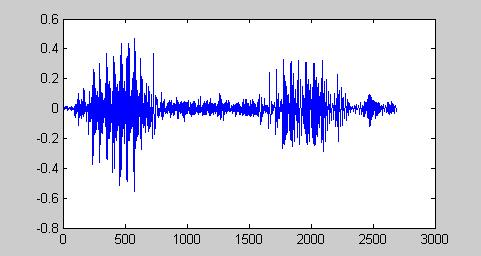刚才的apple.wave这个声音时间才是0.33675秒长语音，但数据量还是巨大的。所以语音信号做各种处理时不是把整个x去做运算，而是把他分割固定的一小段一小段的样子，叫做帧。帧应该多长呢？通常用20ms作为一个帧长。那20ms中包括多少个采样点呢，这个和采样速率fs有关。T=1/fs是采样间隔，那么一帧的数据长度 N=帧时间长度/T=帧时间长度(单位秒)*fs(单位Hz)
上例子中 N=(20ms/1000)*8000=160
分帧后的效果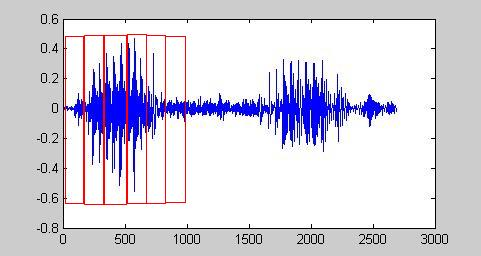上图中帧和帧是挨着的，实际中通常让他们有一定的重贴，这样帧序列可以更好的反应语音信号特点。
接下来语音信号处理都是针对每个帧来处理了，而且帧长是固定，这样处理起来比较方面的 。上例子可以一帧数据包括160个点，

Fs = 8000;
framesize = 80;%真长
overlap = 20;%帧课重叠部分长度
frames = enframe(x, hamming(framesize), overlap);
>> size(frames)
131    80

这样刚才的语音就分成了131个帧了，每帧包含80数据。
80个数据点对于我们来说也比较多的，能不能再进一步减少呢？当然可以这就是特征提取了。现在语音识别中常用的特征是MFCC（可以参照：http://blog.csdn.net/xiaoding133/article/details/8106672）。我们需要知道80个数据点最后提取了多长的特征呢？答案是12个点。
这样语音数据就被进一步缩小了变成131个帧，每帧12点数据了。这样有2个好处：（1）运算量降低 （2）特征比原始语音有更好的分辨能力。到此语音数据的处理部分就可以告一段落了。接下来的问题是这些语音数据和HMM模型怎么关联起来呢？

HMM模型

HMM模型的基本知识一定要读A tutorial on Hidden Markov Models and selected applications in speech recognition, L. Rabiner, 1989, Proc. IEEE 77(2):257–286. 相信我这个英文写的HMM说明比很多中文网站里写的要好懂很多。这里假设大家都懂了HMM模型是什么，我主要说一下，HMM模型怎么用到语音识别的吧。
先从最简单的少词汇量的识别情况说起。

少词汇量语音识别

假设我们要识别的词汇只有 ‘apple’ ‘banana’ ‘kiwi’ ‘lime’ ‘orange’ ‘peach’ ‘pineapple’ 这7个单词。那假设找了15个人，每人说一遍这8个单词然后录音，分别保存成apple01.wav apple02.wav等。这样就得到了每次词15个录音，总共105个wav文件。这就是原始数据。这些数据需要按前面所说分辨成MFCC特征文件。

在少词汇量识别中可以为每个词汇建立一个HMM模型。这个例子中可以建立7个HMM。HMM模型描述包括：

• 状态个数N：整数
• 转移概率A：N×N矩阵
• 初始概率π：N×1矩阵
• 观察序列概率B：N×T矩阵 T是观察符号集个数
• 观察符号：模型输出的东西，可以是数值也可以是向量

其中模型参数B有很多讲究。如果是离散HMM那么B就是个每个状态下可能产生观察符号的概率，对于连续HMM就不能用离散概率来描述输出符号了。举一个例子，论文中有掷骰子的例子，这个HMM的观察符号是离散的1、2、3、4、5、6中的一个。他们观察的情况可以用概率来描述。现在我举一个连续HMM的例子，我有2个小球，一个红色，一个绿色，他们的重量不一样。有一个盒子长度和宽度分别是100cm。最开始我随机取一个球扔进盒子里，等球最后停止运动时记录下它在盒子中的坐标(如下图所示)。然后投掷一个硬币若是正面继续使用刚才的球重复试验，否则换另一个球重复试验。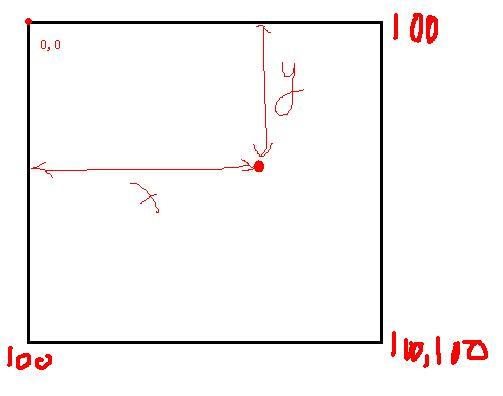为这个过程建立一个HMM模型来描述。其中
N=2分别代表目前用的是红球还是绿球
π=[0.5 0.5] 试验开始我随机取了一个球因此取到红球或绿球的概率一样
A=[
0.8 0.2
0.2 0.8
] 假设我用的硬币质量不均匀，出正面的概率是0.8 背面是0.2
O={x,y} 把球落入盒子的坐标作为观察序列。
假设第一个试验得到的坐标是 {10.5 38.8}，第二次{76.5 18.3}依此类推。现在的问题是HMM中的B矩阵怎么描述？显然我的观察序列可能出现的坐标有无穷多种(假设是实数坐标)，所以离散HMM无法试用了，因为无法穷举我所有的可能的输出。那怎么办呢？可以把观察符号集可以看做是服从连续分布的二维随机变量。连续变量只能用概率密度函数去描述了，而直接说取某个值的概率是无意义的(因为都是无穷小)。我们最熟知分布有：均匀分布、高斯分布等等。那刚才的例子中观察符号的出现服从什么分布？显然我们不知道具体分布。那有没有近似的方法呢？有，那就是混合高斯模型GMM(参照http://blog.pluskid.org/?p=39)。先简化一个，假设观察序列符合高斯分布，那问题是这个假设的高斯分布的均值和方差是多少呢？只要知道这2个参数我们就知道了这个实验的观察符号的服从规律了。那我们假设是不是合理呢？通常情况下自然界很多事情都服从高斯分布，如果这例子本来就不是高斯分布这时我们无法用某个我们已知的概率分布去描述，这时可以用多个高斯分布的线性叠加来描述，这既是GMM。
接下来说一下HMM的隐含性。刚才的例子我已经告诉了N，A，π这些参数，但如果我不告诉你这些参数，而我再幕布后做了这个实验(当然我自己知道，你现在不知道)做了10000次，得到了10000个观察符号，就是观察序列O。然后我把这个O给你，你能不能把我用的N，A，π，观察符号概率分布参数 预测出来？这就是HMM的训练问题。当然这实验是我设计的，所以我是已经知道了各个参数的，你不知道。但很多自然界中的情况我们是提前不能知道参数，而只能看到输出序列，所以我们需要找到系参数让他最能符合这个输出序列（EM算法）。上面的例子如果我不知道参数，只知道观察序列，那么可以这么做，先假设系统的各个参数，然后不停的改变参数让P（O|λ）最大。其中λ={π A μ Σ} 其中{μ Σ}值假设输出时连续单高斯分布时的均值和协方差(多为输出时对应均值向量和协方差矩阵)。
说了很多，再回到语音识别的问题上。要识别7个单词，那就建立7个HMM，那第一个问题HMM的状态用几个？这个需要根据情况来定，看看状态代表什么含义。在整词建模中我们可以认为每个状态代表一个音素，比如apple(ae p l)就是有3个音素构成，那么给apple的HMM定义3个状态。banana(b ax n aa n ax)由6个音素构成，可以用6个状态HMM建模。为了简单也可以为每个词都建立相同状态数的HMM模型，这时就不能说状态精确代表是音素了，可能对应的半音素或几个音素。第二个HMM的观察序列是什么？用语音数据每一帧的MFCC数据作为一个观察符号，一个语音文件的全部MFCC就是观察序列。显然上例子中我的观察符号是2维向量，语音识别例子里就变成12维向量了，而且是连续形式的。第三个问题状态转移有什么限制？用left-right形式的HMM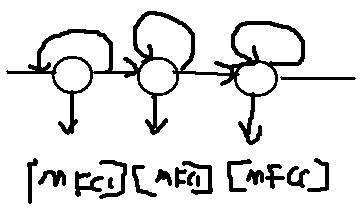这种HMM的A=[
0.2 0.8 0
0 0.2 0.8
0 0 1
] (其中数值时假设的)
这时由语音信号的特点决定的这个类型的HMM。因为如果每个状态代表一个音素，语音一般是一个音素接一个音素这样说，很少出现跳过中间音素，自循环的跳转主要对应发音时声音可能拉长或缩短对应这种情况。
第四个问题每个状态下的观察符号的概率用什么描述？假设单高斯分布（或混合高斯分布，更精确）。
这样一个这个HMM 的定义就有了 λ={N π A μ Σ}
接下来就用各自的观察序列训练各自的模型(就是找到最优的π A μ Σ的问题)。
接下来说一下识别方法。假设有了一段语音那他们到底是哪个单词的发音呢？先把语音分帧变成MFCC序列O，然后分别计算
P（O|λ_apple）
P（O|λ_banana）
P（O|λ_kiwi）
P（O|λ_lime）
P（O|λ_orange）
P（O|λ_peach）
P（O|λ_pineapple）
取最大值作为识别结果输出。这个计算时HMM的第一个问题，可以用前向后项算法计算。
致词希望我把有限词汇量的问题说清楚了，具体算法层面的细节需要仔细阅读上面给出的参考论文。

连续大词汇量语音识别

HMM模型

给每个词单独建立一个HMM的思路在连续大词汇量语音识别中显然不再试用了（词语太多了，而且连续说话时词语的发音会有不同）。那怎么办呢？使识别单元缩小，目前使用较多的是音素
ONE w ah n
YOUNG y ah ng
这时英文单词one,young的音素，其中ah就是他们公共都有的音素了，这样音素真个语音中就比较少了。给出每个单词由哪些音素构成的叫做读音词典，想做语音识别必须先要有读音词典，英文读音词典例子。连续语音识别的基本思想是找出所有音素(数量大概有几十或几百个吧，但要比单词数量少了很多了这就是选择音素的愿意)，然后为每个音素建立一个HMM，然后用各自的音素数据训练各自的HMM模型。
其中HMM模型和语音对应的关系和单个词时的一样，同样用每帧的MFCC特征作为HMM的观察符号（帧是最小单位，比如one这个词的发音包含3个音素，one的wav数据可以分成几百个帧，所以一个音素可以包含多个帧）HMM状态数通常用5个，其中第一个和最后一个状态没有实际意义，中间三个状态分别代表音素开始阶段、音素稳定阶段、音素结束阶段，这三个状态才发射观察序列，头和尾的状态不发射，头和尾状态其他音素连接时一般要去掉的。如下图所示一个音素模型的HMM结构：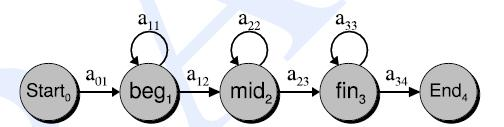这样就能碰另一个问题，一个发音数据中我们怎么知道每个音素从哪儿开始到哪儿结束呢？这叫做音素标注问题。现在有2种做法一种是HMM训练用已经标注的wav文件，另一种是不需要标注也能训练HMM。所谓标准一般需要人工进行，需要听声音，然后判断每个音素的边界。比如one为例0~80ms是发音“w”，81~136ms是发音”ah”,136~536ms是“n”。英文里有timit语料库，有音素标准文件。当然这个标注会非常耗费人力的工作，现在也有语音无需标准就能训练HMM的办法的叫做embedded training （嵌入式训练） ，只需给出语音文件和音素序列就可以比如 语音文件是one.wav 音素信息是“w ah n”，而不用详细给出音素分界线。
有了音素以后就可以用串联音素模型构成字的HMM模型(当然要借助发音词典)。比如英文SIX（s ih k s）由4个音素组成，每个音素又由3状态的HMM构成（连接时头和尾状态要去掉），那么整个SIX的HMM就下图所示(Sb代表第一个音素‘s’的开始阶段的状态)：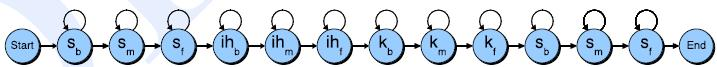同理如果有一个句子“call nine one one”，同样可以为它建立HMM模型（先用音素串联构成词，词串联构成句子）。这个句子的音素构成是“k ao l n ay n w ah n w ah n”，总共有12个音素。句子的HMM的A矩阵和B矩阵是有各个音素HMM的A矩阵和B矩阵构成的。比如音素“k”HMM定义的A矩阵如下：
A_k=[
0 1 0 0 0
0 1/2 1/2 0 0
0 0 1/2 1/2 0
0 0 0 1/2 1/2
0 0 0 0 0
]

A_ao=[
0 1 0 0 0
0 1/2 1/2 0 0
0 0 1/2 1/2 0
0 0 0 1/2 1/2
0 0 0 0 0
]

A_l=[
0 1 0 0 0
0 1/2 1/2 0 0
0 0 1/2 1/2 0
0 0 0 1/2 1/2
0 0 0 0 0
]

那”call”的HMM的A矩阵就是
A_call=[
0 1 0 0 0 0 0 0 0 0 0
0 1/2 1/2 0 0 0 0 0 0 0 0
0 0 1/2 1/2 0 0 0 0 0 0 0
0 0 0 1/2 1/2 0 0 0 0 0 0
0 0 0 0 1/2 1/2 0 0 0 0 0
0 0 0 0 0 1/2 1/2 0 0 0 0
0 0 0 0 0 0 1/2 1/2 0 0 0
0 0 0 0 0 0 0 1/2 1/2 0 0
0 0 0 0 0 0 0 0 1/2 1/2 0
0 0 0 0 0 0 0 0 0 1/2 1/2
0 0 0 0 0 0 0 0 0 0 0
]
句子以此类推。A矩阵中和多地方都是0，代表不可能的状态转移。句子中的B矩阵和音素的B矩阵一样，但一个句子音素可能出现多次，因此B矩阵是可以共享的。

Embedded Training

我们的目的是训练各个音素的HMM模型参数，用这个HMM模型去代表语音的音素。最简单的训练方式是我们有很多音素对应的观察序列，然后用Baum-Welch算法(EM算法的一个实现方式)去训练A、B矩阵。但前面说过我们很难从一个句子或词语的发音中精确的找到音素、甚至是伴音素的边界。为了解决这个苦难一般采用embedded training的方式。embedded training需要的数据包括：
①训练用的句子的文本文件、例子： call nine one one !
②读音词典。例子：
CALL k ao l
NINE n ay n
ONE w ah n
③语音文件。sen01.wav sen02.wav
④音素列表级音素原始HMM定义
embedded training的基本思路是：读取一个句子的文本、把文本表示的一个句子转成用音素表示的句子(利用读音词典)、利用原始音素HMM定义串联起来构成句子的HMM定义(可能是非常长的HMM了)。然后把一个句子的wav文件转换成帧、提取特征，变成长的特征序列。然后把整个句子的特征序列看做是句子HMM模型的观察序列，直接却训练长的句子HMM模型(这时和普通的HMM模型一样用Baum-Welch算法)。句子训练好以后实际上各个音素也训练好了(因为句子的A、B矩阵就由音素的A、B矩阵构成)。看下图：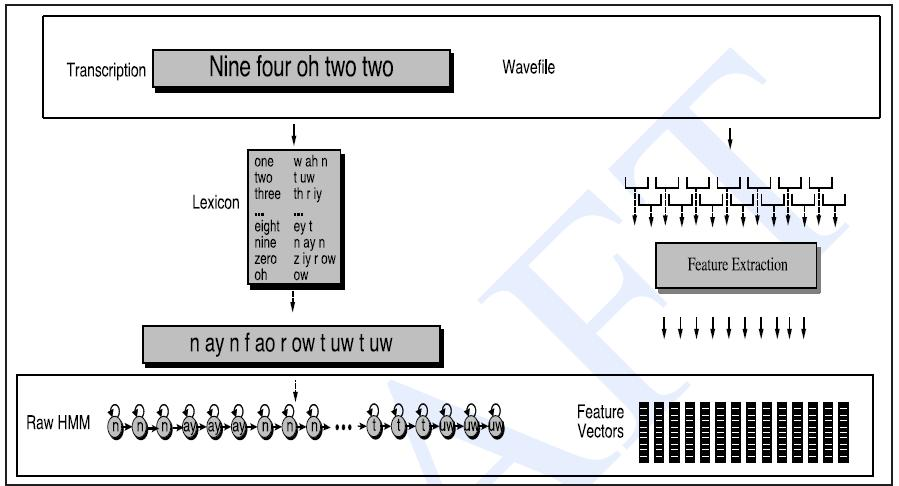算法步骤如下：
1.为每个训练句子建立整句HMM模型
2.初始化整句HMM模型的A矩阵，其中开始和结束状态外，每个状态只能到自己或下一个状态，概率分别是0.5
3.所有状态的B矩阵(一般用混合高斯模型)用全部训练样本的均值和方差初始化高斯模型的均值和方差
4.多次执行Baum-Welch算法。

语音解码

如上所属如果所有音素的HMM都训练好了，那么现在来了一个声音文件、怎么转换成文本呢？这就是语音解码问题。“给定一个音素模型的观察序列，和他对应的概率最大的文字序列是什么？”这就是解码问题。

观察序列 O=o1,o2,o3,...,ot,
文本序列 W=w1,w2,w3,...,wt,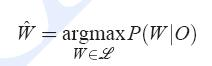识别就是在给定观察序列(代表的是声音信息)下，在所有的文字序列集合中哪个的概率最高的问题。把概率最高的文字序列看做是语音识别的结果。计算P(W|O)的难度比较大，所以这里通过贝叶斯公式进行转换如下：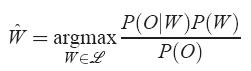其中P(W)是文字先验概率（N-gram模型可以描述），P(O|W)是已知文字下获得观察序列O的概率叫做声学模型(HMM模型可以描述)。P（O）在分母上，对所有的W都是一样的所以可以忽略不用计算。那就变成：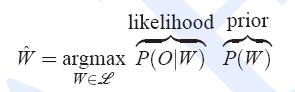这两项概率一般需要做一些权重的修正，实际中经常使用：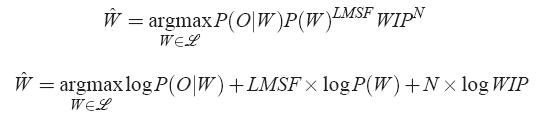第二个公式取对数后的形式(计算一般用对数概率)，其中LMSF是语言模型的放大倍数同行取5~15之间的数，N：文字长度，WIP是常数叫做word insertion penalty

连续语音识别中解码的难点：
- 连续语音中词与词的边界是不知道的
- 给出一段语音信号，其中包含的额文字个数是未知的
- 搜索全部可能性是很难的，比如总共有M个词，语音长度是V个帧，全部组合是 Mv 这么多可能性，无法全部遍历。

解码的基本思路：
用训练好的音素模型为每个字构建HMM模型(字个数应该包含目标语种中全部或常用的字)。其中字与字之间的关系用语言模型来描述(N-gram)。字和发音序列之间的关系用HMM模型来描述。利用这两部分信息构建一个庞大的网状结构(具体实现形式可以有很多种)叫做搜索空间，然后在这个上运行搜索算法（有Viterbi算法、Viterbi with Beam-Pruning、Token传递算法等等）获得最优可能的文字序列作为识别结果。

其中搜索空间可以看成是三层结构：网络层、字层、HMM层。如图所示：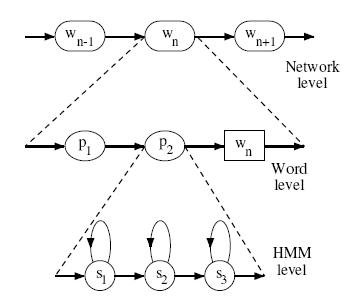对未知的语音序列，假设有T个帧构成。从识别网络所有可能入口开始经过T个HMM发射状态都叫做一个识别路径。每个路径都可以计算一个对数概率值。每个路径可能包含 音素HMM状态之间的转移、音素边界之间的转移和字边界之间的转移三部分。HMM内部概率可以由HMM模型计算、音素边界可以用固定概率、字边界转移概率可以用语言模型的N-gram概率提前获得。
Token Passing Algorithm
每个Token代表识别网络中的一个路径，内部存储该条路径的概率和回溯信息。t=0时刻，每个可能的开始节点(对应于音素HMM的状态)生成一个token。每经过1帧(t=t+1)token向所有可能的下一个节点传播(当前节点和多个节点有连接时token复制)。每次转播到发射节点(音素边界、字边界也看做是节点但不发射观察序列)时暂停，然后token里记录的对数概率增加(参考转移概率A和观察序列矩阵B)，然后再传播。可以规定每个节点最多同时保持N个token（最简单情况N=1，保留多个token中概率最高的一个进行传播，或者采取一些剪枝策略(purning)）。其中token穿过字边界时可以把N-gram模型的概率加入到token的对数概率里，这样就综合考虑和音素模型和语言模型了。同时穿过字边界时需要记录路径，最后结束时用回溯列出识别文字（从这一点上看识别网络中不存的字肯定是无法识别的了）。

三音素模型

所有上述内容都用单音素模型了，实际中通常使用三音素模型(Triphone)。需要考虑上下音素的协同发音情况。例子：
单音素：BRYAN → B R AY AX N
三音素：BRYAN → SIL-B+R B-R+AY R-AY+AX AY-AX+N AX-N+SIL
其中“-”代表当前音素，“+”代表后续音素，“-”号前的代表前面的音素。三音素的好处就是音素级别建模更精确了，不如本来有50个音素，单因素只需要训练50个HMM，三音素就出现 503=12500 个HMM需要训练了。参数就会变得巨大，相比训练数据就会稀疏很多了。解决方案就是：三音素合并、状态共享等。并不是所有的三音素都合法、其次有些三音素很接近利用一些聚类的方式可以合并三音素减少模型数量。其次观察矩阵B对于很多音素出现再不同的三音素里可能是差不多的，这时就可以共享同一个B矩阵了（详细省略）。
至此原理部分基本讲完了，希望大家能看懂
主要用的资料有：

HMM基础论http://www.cs.ubc.ca/~murphyk/Software/HMM/rabiner.pdf
HTK说明文档 htkbook.pdf （网上可下载）
【Speech and Language Processing】Daniel Jurafsky & James H. Martin 提取码：ceye

本文中有些图是摘自上述资料。

全部结束感谢大家的阅读！

展开全文• HMM识别4中方言，每种方言80个作为训练，40个作为识别。MATLAB代码。HMM语音识别
• 该压缩包中包含一个完整的语音识别程序，代码使用matlab实现，使用了经典的GMMHMM模型。附件中还包含完整的说明文档，介绍了一些基本原理，和该源码的使用方法。是语音识别入门必读源码之一。该源码只需要很小的...
• 为了对GMM-HMM在语音识别上的应用有个宏观认识，花了些时间读了下HTK（用htk完成简单的孤立词识别）的部分源码，对该算法总算有了点大概认识，达到了预期我想要的。不得不说，网络上关于语音识别的通俗易懂教程太少...

为了对GMM-HMM在语音识别上的应用有个宏观认识，花了些时间读了下HTK（用htk完成简单的孤立词识别）的部分源码，对该算法总算有了点大概认识，达到了预期我想要的。不得不说，网络上关于语音识别的通俗易懂教程太少，都是各种公式满天飞，很少有说具体细节的，当然了，那需要有实战经验才行。下面总结以下几点，对其有个宏观印象即可（以孤立词识别为例）。

一、每个单词的读音都对应一个HMM模型，大家都知道HMM模型中有个状态集S，那么每个状态用什么来表示呢，数字？向量？矩阵？其实这个状态集中的状态没有具体的数学要求，只是一个名称而已，你可以用’1’, ’2’, ‘3’…表示，也可以用’a’, ‘b’, ’c ’表示。另外每个HMM模型中到底该用多少个状态，是通过先验知识人为设定的。

二、HMM的每一个状态都对应有一个观察值，这个观察值可以是一个实数，也可以是个向量，且每个状态对应的观察值的维度应该相同。假设现在有一个单词的音频文件，首先需要将其进行采样得到数字信息（A/D转换），然后分帧进行MFCC特征提取，假设每一帧音频对应的MFCC特征长度为39，则每个音频文件就转换成了N个MFCC向量（不同音频文件对应的N可能不同），这就成了一个序列，而在训练HMM模型的参数时（比如用Baum-Welch算法），每次输入到HMM中的数据要求就是一个观测值序列。这时，每个状态对应的观测值为39维的向量，因为向量中元素的取值是连续的，需要用多维密度函数来模拟，通常情况下用的是多维高斯函数。在GMM-HMM体系中，这个拟合函数是用K个多维高斯混合得到的。假设知道了每个状态对应的K个多维高斯的所有参数，则该GMM生成该状态上某一个观察向量（一帧音频的MFCC系数）的概率就可以求出来了。

三、对每个单词建立一个HMM模型，需要用到该单词的训练样本，这些训练样本是提前标注好的，即每个样本对应一段音频，该音频只包含这个单词的读音。当有了该单词的多个训练样本后，就用这些样本结合Baum-Welch算法和EM算法来训练出GMM-HMM的所有参数，这些参数包括初始状态的概率向量，状态之间的转移矩阵，每个状态对应的观察矩阵（这里对应的是GMM，即每个状态对应的K个高斯的权值，每个高斯的均值向量和方差矩阵）。

四、在识别阶段，输入一段音频，如果该音频含有多个单词，则可以手动先将其分割开（考虑的是最简单的方法），然后提取每个单词的音频MFCC特征序列，将该序列输入到每个HMM模型（已提前训练好的）中，采用前向算法求出每个HMM模型生成该序列的概率，最后取最大概率对应的那个模型，而那个模型所表示的单词就是我们识别的结果。

五、在建立声学模型时，可以用Deep Learning的方法来代替GMM-HMM中的GMM，因为GMM模拟任意函数的功能取决于混合高斯函数的个数，所以具有一定的局限性，属于浅层模型。而Deep Network可以模拟任意的函数，因而表达能力更强。注意，这里用来代替GMM的Deep Nets模型要求是产生式模型，比如DBN，DBM等，因为在训练HMM-DL网络时，需要用到HMM的某个状态产生一个样本的概率。

六、GMM-HMM在具体实现起来还是相当复杂的。

七、一般涉及到时间序列时才会使用HMM，比如这里音频中的语音识别，视频中的行为识别等。如果我们用GMM-HMM对静态的图片分类，因为这里没涉及到时间信息，所以HMM的状态数可设为1，那么此时的GMM-HMM算法就退化成GMM算法了。

MFCC:

MFCC的matlab实现教程可参考：张智星老师的网页教程mfcc. 最基本的12维特征。

function mfcc=frame2mfcc(frame, fs, filterNum, mfccNum, plotOpt)
% frame2mfcc: Frame to MFCC conversion.
%    Usage: mfcc=frame2mfcc(frame, fs, filterNum, mfccNum, plotOpt)
%
%    For example:
%        waveFile='what_movies_have_you_seen_recently.wav';
%        startIndex=12000;
%        frameSize=512;
%        frame=y(startIndex:startIndex+frameSize-1);
%        frame2mfcc(frame, fs, 20, 12, 1);

%    Roger Jang 20060417

if nargin<1, selfdemo; return; end
if nargin<2, fs=16000; end
if nargin<3, filterNum=20; end
if nargin<4, mfccNum=12; end
if nargin<5, plotOpt=0; end

frameSize=length(frame);
% ====== Preemphasis should be done at wave level
%a=0.95;
%frame2 = filter([1, -a], 1, frame);
frame2=frame;
% ====== Hamming windowing
frame3=frame2.*hamming(frameSize);
% ====== FFT
[fftMag, fftPhase, fftFreq, fftPowerDb]=fftOneSide(frame3, fs);
% ====== Triangular band-pass filter bank
triFilterBankPrm=getTriFilterBankPrm(fs, filterNum);    % Get parameters for triangular band-pass filter bank
% Triangular bandpass filter.
for i=1:filterNum
tbfCoef(i)=dot(fftPowerDb, trimf(fftFreq, triFilterBankPrm(:,i)));%得到filterNum个滤波系数
end
% ====== DCT
mfcc=zeros(mfccNum, 1); %DCT变换的前后个数也没有变
for i=1:mfccNum
coef = cos((pi/filterNum)*i*((1:filterNum)-0.5))'; %mfcc中的前mfccNum个系数
mfcc(i) = sum(coef.*tbfCoef');%直接按照DCT公式
end
% ====== Log energy
%logEnergy=10*log10(sum(frame.*frame));
%mfcc=[logEnergy; mfcc];

if plotOpt
subplot(2,1,1);
plot(frame, '.-');
set(gca, 'xlim', [-inf inf]);
title('Input frame');
subplot(2,1,2);
plot(mfcc, '.-');
set(gca, 'xlim', [-inf inf]);
title('MFCC vector');
end

% ====== trimf.m (from fuzzy toolbox)
function y = trimf(x, prm) %由频率的横坐标算出三角形内的纵坐标,0~1
a = prm(1); b = prm(2); c = prm(3);
y = zeros(size(x));
% Left and right shoulders (y = 0)
index = find(x <= a | c <= x);
y(index) = zeros(size(index)); %只考虑三角波内的量
% Left slope
if (a ~= b)
index = find(a < x & x < b);
y(index) = (x(index)-a)/(b-a);
end
% right slope
if (b ~= c)
index = find(b < x & x < c);
y(index) = (c-x(index))/(c-b);
end
% Center (y = 1)
index = find(x == b);
y(index) = ones(size(index));

% ====== Self demo
function selfdemo
waveFile='what_movies_have_you_seen_recently.wav';
startIndex=12000;
frameSize=512;
frame=y(startIndex:startIndex+frameSize-1);
feval(mfilename, frame, fs, 20, 12, 1);

ZCR:

过0检测，用于判断每一帧中过零点的数量情况，最简单的版本可参考：zeros cross rate.

waveFile='csNthu.wav';
frameSize=256;
overlap=0;
frameMat=enframe(y, frameSize, overlap);
frameMat(:,i)=frameMat(:,i)-mean(frameMat(:,i));    % mean justification
end
zcr=sum(frameMat(1:end-1, :).*frameMat(2:end, :)<0);
sampleTime=(1:length(y))/fs;
subplot(2,1,1); plot(sampleTime, y); ylabel('Amplitude'); title(waveFile);
subplot(2,1,2); plot(frameTime, zcr, '.-');
xlabel('Time (sec)'); ylabel('Count'); title('ZCR');

EPD:

端点检测，检测声音的起始点和终止点，可参考：EPD in Time Domain,在时域中的最简单检测方法。

waveFile='sunday.wav';
frameSize = 256;
overlap = 128;

wave=wave-mean(wave);                % zero-mean substraction
frameMat=buffer2(wave, frameSize, overlap);    % frame blocking,每一列代表一帧
frameNum=size(frameMat, 2);            % no. of frames
volume=frame2volume(frameMat);        % volume,求每一帧的能量，绝对值或者平方和,volume为行向量
volumeTh1=max(volume)*0.1;            % volume threshold 1
volumeTh2=median(volume)*0.1;            % volume threshold 2
volumeTh3=min(volume)*10;            % volume threshold 3
volumeTh4=volume(1)*5;                % volume threshold 4
index1 = find(volume>volumeTh1); %找出volume大于阈值的那些帧序号
index2 = find(volume>volumeTh2);
index3 = find(volume>volumeTh3);
index4 = find(volume>volumeTh4);
%frame2sampleIndex()为从帧序号找到样本点的序号(即每一个采样点的序号)
%endPointX长度为2,包含了起点和终点的样本点序号
endPoint1=frame2sampleIndex([index1(1), index1(end)], frameSize, overlap);
endPoint2=frame2sampleIndex([index2(1), index2(end)], frameSize, overlap);
endPoint3=frame2sampleIndex([index3(1), index3(end)], frameSize, overlap);
endPoint4=frame2sampleIndex([index4(1), index4(end)], frameSize, overlap);

subplot(2,1,1);
time=(1:length(wave))/fs;
plot(time, wave);
ylabel('Amplitude'); title('Waveform');
axis([-inf inf -1 1]);
line(time(endPoint1(  1))*[1 1], [-1, 1], 'color', 'm');%标起点终点线
line(time(endPoint2(  1))*[1 1], [-1, 1], 'color', 'g');
line(time(endPoint3(  1))*[1 1], [-1, 1], 'color', 'k');
line(time(endPoint4(  1))*[1 1], [-1, 1], 'color', 'r');
line(time(endPoint1(end))*[1 1], [-1, 1], 'color', 'm');
line(time(endPoint2(end))*[1 1], [-1, 1], 'color', 'g');
line(time(endPoint3(end))*[1 1], [-1, 1], 'color', 'k');
line(time(endPoint4(end))*[1 1], [-1, 1], 'color', 'r');
legend('Waveform', 'Boundaries by threshold 1', 'Boundaries by threshold 2', 'Boundaries by threshold 3', 'Boundaries by threshold 4');

subplot(2,1,2);
plot(frameTime, volume, '.-');
ylabel('Sum of Abs.'); title('Volume');
axis tight;
line([min(frameTime), max(frameTime)], volumeTh1*[1 1], 'color', 'm');
line([min(frameTime), max(frameTime)], volumeTh2*[1 1], 'color', 'g');
line([min(frameTime), max(frameTime)], volumeTh3*[1 1], 'color', 'k');
line([min(frameTime), max(frameTime)], volumeTh4*[1 1], 'color', 'r');
legend('Volume', 'Threshold 1', 'Threshold 2', 'Threshold 3', 'Threshold 4');

GMM:

GMM用在拟合数据分布上，本质上是先假设样本的概率分布为GMM，然后用多个样本去学习这些GMM的参数。GMM建模在语音中可用于某个单词的发音，某个人的音色等。其训练过程可参考:speaker recognition.

function [M, V, W, logProb] = gmmTrain(data, gaussianNum, dispOpt)
% gmmTrain: Parameter training for gaussian mixture model (GMM)
%    Usage: function [M, V, W, logProb] = gmm(data, gaussianNum, dispOpt)
%        data: dim x dataNum matrix where each column is a data point
%        gaussianNum: No. of Gaussians or initial centers
%        dispOpt: Option for displaying info during training
%        M: dim x meanNum matrix where each column is a mean vector
%        V: 1 x gaussianNum vector where each element is a variance for a Gaussian
%        W: 1 x gaussianNum vector where each element is a weighting factor for a Gaussian

% Roger Jang 20000610

if nargin==0, selfdemo; return; end
if nargin<3, dispOpt=0; end

maxLoopCount = 50;    % Max. iteration
minImprove = 1e-6;    % Min. improvement
minVariance = 1e-6;    % Min. variance
logProb = zeros(maxLoopCount, 1);   % Array for objective function
[dim, dataNum] = size(data);

% Set initial parameters
% Set initial M
%M = data(1+floor(rand(gaussianNum,1)*dataNum),:);    % Randomly select several data points as the centers
if length(gaussianNum)==1,
% Using vqKmeans to find initial centers
fprintf('Start KMEANS to find the initial mu...\n');
%    M = vqKmeansMex(data, gaussianNum, 0);
M = vqKmeans(data, gaussianNum, 0); %利用聚类的方法求均值,聚成gaussianNum类
%    M = vqLBG(data, gaussianNum, 0);
fprintf('Start GMM training...\n');
if any(any(~isfinite(M))); keyboard; end
else
% gaussianNum is in fact the initial centers
M = gaussianNum;
gaussianNum = size(M, 2);
end
% Set initial V as the distance to the nearest center
if gaussianNum==1
V=1;
else
distance=pairwiseSqrDist(M);%pairwiseSqrDist是dll
%distance=pairwiseSqrDist2(M);

distance(1:(gaussianNum+1):gaussianNum^2)=inf;    % Diagonal elements are inf
[V, index]=min(distance);    % Initial variance for each Gaussian
end
% Set initial W
W = ones(1, gaussianNum)/gaussianNum;    % Weight for each Gaussian,初始化时是均分权值

if dispOpt & dim==2, displayGmm(M, V, data); end
for i = 1:maxLoopCount  %开始迭代训练参数,EM算法
% Expectation step:
% P(i,j) is the probability of data(:,j) to the i-th Gaussian
% Prob为每个样本在GMM下的概率
[prob, P]=gmmEval(data, M, V, W);
logProb(i)=sum(log(prob)); %所有样本的联合概率
if dispOpt
fprintf('i = %d, log prob. = %f\n',i-1, logProb(i));
end
PW = diag(W)*P;
BETA=PW./(ones(gaussianNum,1)*sum(PW));    % BETA(i,j) is beta_i(x_j)
sumBETA=sum(BETA,2);

% Maximization step:  eqns (2.96) to (2.98) from Bishop p.67:
M = (data*BETA')./(ones(dim,1)*sumBETA');

DISTSQ = pairwiseSqrDist(M, data);                    % Distance of M to data
%DISTSQ = pairwiseSqrDist2(M, data);                    % Distance of M to data

V = max((sum(BETA.*DISTSQ, 2)./sumBETA)/dim, minVariance);    % (2.97)
W = (1/dataNum)*sumBETA;                    % (2.98)

if dispOpt & dim==2, displayGmm(M, V, data); end
if i>1, if logProb(i)-logProb(i-1)<minImprove, break; end; end
end
[prob, P]=gmmEval(data, M, V, W);
logProb(i)=sum(log(prob));
fprintf('Iteration count = %d, log prob. = %f\n',i, logProb(i));
logProb(i+1:maxLoopCount) = [];

% ====== Self Demo ======
function selfdemo
%[data, gaussianNum] = dcdata(2);
data = rand(1000,2);
gaussianNum = 8;
data=data';
plotOpt=1;
[M, V, W, lp] = feval(mfilename, data, gaussianNum, plotOpt);

pointNum = 40;
x = linspace(min(data(1,:)), max(data(1,:)), pointNum);
y = linspace(min(data(2,:)), max(data(2,:)), pointNum);
[xx, yy] = meshgrid(x, y);
data = [xx(:) yy(:)]';
z = gmmEval(data, M, V, W);
zz = reshape(z, pointNum, pointNum);
figure; mesh(xx, yy, zz); axis tight; box on; rotate3d on
figure; contour(xx, yy, zz, 30); axis image

% ====== Other subfunctions ======
function displayGmm(M, V, data)
% Display function for EM algorithm
figureH=findobj(0, 'tag', mfilename);
if isempty(figureH)
figureH=figure;
set(figureH, 'tag', mfilename);
colordef black
plot(data(1,:), data(2,:),'.r'); axis image
theta=linspace(-pi, pi, 21);
x=cos(theta); y=sin(theta);
sigma=sqrt(V);
for i=1:length(sigma)
circleH(i)=line(x*sigma(i)+M(1,i), y*sigma(i)+M(2,i), 'color', 'y');
end
set(circleH, 'tag', 'circleH', 'erasemode', 'xor');
else
circleH=findobj(figureH, 'tag', 'circleH');
theta=linspace(-pi, pi, 21);
x=cos(theta); y=sin(theta);
sigma=sqrt(V);
for i=1:length(sigma)
set(circleH(i), 'xdata', x*sigma(i)+M(1,i), 'ydata', y*sigma(i)+M(2,i));
end
drawnow
end

Speaker identification:

给N个人的语音资料，用GMM可以训练这N个人的声音模型，然后给定一段语音，判断该语音与这N个人中哪个最相似。方法是求出该语音在N个GMM模型下的概率，选出概率最大的那个。可参考:speaker recognition.

function [recogRate, confusionMatrix, speakerData]=speakerIdentify(speakerData, speakerGmm, useIntGmm)
% speakerIdentify: speaker identification using GMM parameters
%    Usage: [recogRate, confusionMatrix, speakerData]=speakerIdentify(speakerData, speakerGmm, useIntGmm)
%        speakerData: structure array generated by speakerDataRead.m
%        speakerGmm: speakerGmm(i).gmmPrm is the GMM parameters for speaker i.
%        useIntGmm: use fixed-point GMM

%    Roger Jang, 20070517, 20080726

if nargin<3, useIntGmm=0; end

% ====== Speaker identification using GMM parameters
speakerNum=length(speakerData);
for i=1:speakerNum
%    fprintf('%d/%d: Recognizing wave files by %s\n', i, speakerNum, speakerData(i).name);
for j=1:length(speakerData(i).sentence)
%        fprintf('\tSentece %d...\n', j);
%找出一个句子，看它属于哪个speaker
for k=1:speakerNum,
%            fprintf('\t\tSpeaker %d...\n', k);
%    logProb(k, :)=gmmEval(speakerData(i).sentence(j).fea, speakerGmm(k).gmmPrm);
if ~useIntGmm
%    logProb(k, :)=gmmEvalMex(speakerData(i).sentence(j).fea, gmm(k).mean, gmm(k).covariance, gmm(k).weight);
logProb(k, :)=gmmEval(speakerData(i).sentence(j).fea, speakerGmm(k).gmmPrm);
else
%    logProb(k, :)=gmmEvalIntMex(speakerData(i).sentence(j).fea, gmm(k).mean, gmm(k).covariance, gmm(k).weight);
logProb(k, :)=gmmEvalIntMex(speakerData(i).sentence(j).fea, speakerGmm(i).gmmPrm);
end
end
cumLogProb=sum(logProb, 2);
[maxProb, index]=max(cumLogProb);
speakerData(i).sentence(j).predictedSpeaker=index; %找出身份
speakerData(i).sentence(j).logProb=logProb;
end
end

% ====== Compute confusion matrix and recognition rate
confusionMatrix=zeros(speakerNum);
for i=1:speakerNum,
predictedSpeaker=[speakerData(i).sentence.predictedSpeaker];
[index, count]=elementCount(predictedSpeaker);
confusionMatrix(i, index)=count;
end
recogRate=sum(diag(confusionMatrix))/sum(sum(confusionMatrix));

GMM-HMM:

训练阶段：给出HMM的k个状态，每个状态下的观察样本的生成可以用一个概率分布来拟合，这里是采用GMM拟合的。其实，可以把GMM-HMM整体看成是一个生成模型。给定该模型的5个初始参数(结合随机和训练样本获得)，启动EM算法的E步：获得训练样本分布，即计算训练样本在各个状态下的概率。M步：用这些训练样本重新评估那5个参数。

测试阶段：(以孤立词识别为例)给定每个词发音的frame矩阵，取出某一个GMM-HMM模型，算出该发音每一帧数据在取出的GMM-HMM模型各个state下的概率，结合模型的转移概率和初始概率，获得对应的clique tree，可用图模型的方法inference出生成该语音的概率。比较多个GMM-HMM模型，取最大概率的模型对应的词。

参考资料：

展开全文机器学习 数据挖掘 笔记 GMM-HMM 语音识别
• 详细介绍了训练hmmgmm的EM算法，以及其应用，对利用这些模型的朋友，想了解此算法的最好的资料。
• 基于MATLABHMM非特定人孤立词语音识别，包括语音库，程序完整可以运行，包括HMM的voicebox的函数库， 点击运行test文件直接运行
• 近年来，音频识别作为...GMM-UBM作为概率统计模型，由于其能够很好地模拟说话人的声学特征分布，实现方法灵活有效，加上具有较高的鲁棒性，故提出后就迅速成为说话人识别中的重要建模方法。一、特征参数的提取对于...

近年来，音频识别作为个人信息验证领域的研究热点发展迅速。目前，语音识别系统在实验室环境中已经可以获得相当好的效果，但在现实场景中，由于噪声的干扰，系统的识别率将受到严重影响，这大大妨碍了语音识别技术在实际环境中的应用。

GMM-UBM作为概率统计模型，由于其能够很好地模拟说话人的声学特征分布，实现方法灵活有效，加上具有较高的鲁棒性，故提出后就迅速成为说话人识别中的重要建模方法。

一、特征参数的提取

对于说话人确认系统来说，从每一帧里提取出代表说话人信息的特征参数是之后所有步骤的基础。在文献中研究了多种特征参数。其中，线性预测参数(Linear Prediction Coefficients, LPC因为其直接由人的发声模型推导而来而受到了广泛关注。知觉预测参数(Perceptual Linear Prediction Coefficients, PLPC)也使用了同样的计算方法。这些参数是基于人体知觉，根据人的听觉过程而开发出来。但是近二十年来，应用最广泛的还是使用傅里叶变换得到的参数。其中由Davies和Mermelstein提出的Mel倒谱系数(MFCC)由于其充分考虑人体知觉原理和较强的鲁棒性，以及在倒谱域的灵活运用，成为了在说话人识别领域使用最广，效果最好的特征参数。本文关于说话人确认的实验全部采用Mel倒谱系数作为特征参数。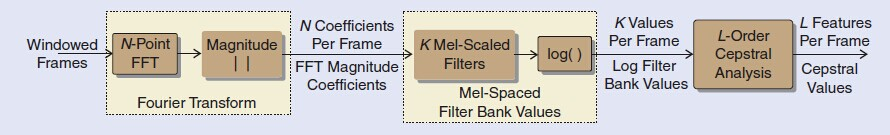图1Mel倒谱系数的计算过程

Fig.1 Analysis block diagram for MFCC feature vectors

在说话人确认中，提取特征参数的一个重要进展是联合MFCC的一阶，二阶甚至三阶导数可以刻画帧间的动态联系。这些动态信息可以更形象地表现出说话人的特征。类似于与文本有关的语音识别中，这些动态联系可以刻画出说话人的表达习惯。如果定义为第t帧，则MFCC的一阶导数可表示为：

P通常取值为2。

同理可得，将换成可以得到二阶导数。按照同样的计算规则可以依次得到MFCC的更高阶导数。

这些高阶MFCC将联合原阶的MFCC一起构成说话人确认的特征参数。举例来说，一个13维的MFCC参数(L=12加上c0)可以得到26维的联合一阶MFCC的特征参数，或39维的同时联合一阶和二阶MFCC的级联特征参数，详细阐释请见图2-5。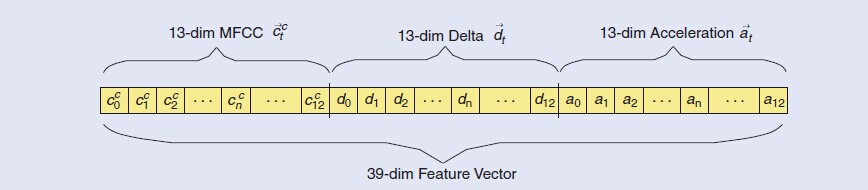图2级联高阶导数的MFCC

Fig.2 Concatenate of MFCC features with temporal derivatives

二、联合背景模型的混合高斯模型

在说话人确认中，验证测试者是否为已经登记过的目标话者，需要将其的测试语音分别在说话人模型(GMM)和背景模型(UBM)中分别打分，以两者的比值作为最后的结果再与先前设置的阈值进行比较。UBM作为一个混合度较大的GMM(M=256或更多)，通过大量说话人的语音数据的训练，平衡了不同人之间发音的差异，可用于任何话者的确认。虽然UBM的训练数据量大幅上升，但由于其表达了与话者无关的特征分布，是所有话者的“并集”，具有背景意义。

GMM-UBM主要用于开集的说话者辨认，因为GMM的性能足以应对普通的闭集测试集合。此外，UBM比单个说话人GMM更精确可靠的原因在于它在训练时调用了所有说话人的数据，因此UBM不会受到训练数据不足以及隐性数据(unseen data)的影响。

根据MAP自适应算法可以对说话人的权重，均值，方差分别进行调整，但一般情况只对均值进行调整，实验表明只调整均值效最佳，其具体公式如下：

其中，代表了调整后的第m个高斯分布函数的均值；是调整时的规整因子，由先验知识得到；代表了话者的训练数据与原本UBM的相似度，越大，说明说话人的模型与UBM越相似；是原本UBM的第m个高斯分布函数的均值；代表了由说话人自身数据训练得到的均值。

由式2-13可知，越大，就越接近1(为定值)，越接近0， 表明话者模型进行修正时只改变与UBM中与说话人特征相似的高斯分布函数，使其更接近于目标话者的特征分布，体现了说话人的个性。反之，越小，就越接近0，越接近1，表明与UBM中与说话人特征相似度不高(即隐性信息)的部分几乎保持不变，这样在计算匹配度比值时就避免了低匹配度情况的出现，即原本的隐性信息被融合于背景模型中。此外，当关于话者个人的训练语音增多时，修正后的话者模型也就越远离原本的背景模型而接近真实话者模型的分布。

使用GMM-UBM的优势在于，因为UBM是由大量说话人的数据训练而成，单独训练每一个说话人模型时只需要少量数据进行修正即可，这样得到的话者GMM模型比原本直接训练GMM要可靠得多。此外，由于GMM-UBM中的GMM拥有与UBM相同多的维数，这比单独训练的GMM的维数要多出许多，正因为借助了UBM的优势，所以GMM-UBM在处理隐性数据方面的效果要比GMM好得多。

在GMM-UBM框架下，测试语音的匹配度计算是测试语音与说话人模型GMM和背景模型UBM匹配输出似然度的比值，在评分取对数的情况下，表现为两者的差值，如式2-14所示。

其中是测试语音的一帧的特征参数，和分别是目标模型和背景模型。由式2-14可知，在匹配度计算时，由于两者相减，使原本说话人模型中与背景模型相似的部分、背景噪音和通道的影响被消除，更加凸显说话人个性的同时，也增强了系统的鲁棒性。

假设有N个说话人，基于GMM-UBM的说话人确认系统的训练和测试的过程如图3所示。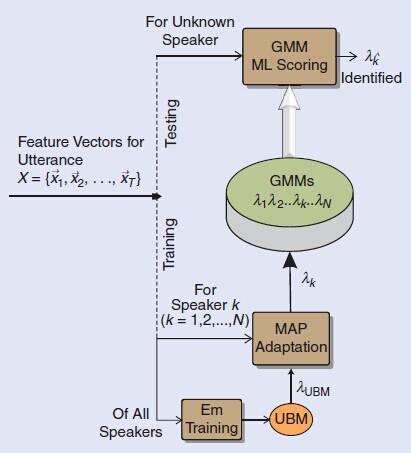图3 GMM-UBM的训练与测试过程

Fig.3 GMM-UBM training and testing framework

三、效果总结

通过分析四种特征参数MFCC、LPC、LFS、LPCC和三种建模方式GMM、HMM、codebook的不同组合对噪声识别的影响，并分别从纯噪声和估计噪声两个方面进行实验。实验结果如图：

测试语句

噪声类型

噪声识别结果

airport

babble

car

exhibition

restaurant

station

street

train

airport

24

0

0

0

0

6

0

0

babble

2

27

0

0

0

1

0

0

car

1

0

29

0

0

0

0

0

exhibition

0

0

0

30

0

0

0

0

restaurant

2

0

0

0

22

6

0

0

station

1

0

0

0

0

29

0

0

street

2

0

0

0

0

5

23

0

train

0

0

0

0

0

0

0

30

实验结果证明MFCC联合GMM的方法是噪声识别中的最佳组合。最后还分析了该方法对不同的噪声类型的识别结果，大多数估计噪声的正确识别率都能达到70%及以上，说明了MFCC和GMM的组合在噪声识别的实际应用中具有一定意义。

展开全文• gmmmatlab代码高斯混合模型 使用Sylvian Calinon的pbdlib存储库为机器人轨迹重新创建GMM / HMM 该资料库包含重建Sylvian Calinon的pbdlib-python资料库[Link：]中演示的GMM / HMM / HSMM模型的过程，以便训练...
• matlab语言写的关于语音识别技术中最为流行的匹配算法hmm,可以实现端点检测和基于DTW和HMM的孤立词识别和连续语音识别
• 高斯混合模型（Gaussian Mixture Model, GMM）是单一高斯概率密度函数的延伸，GMM能够平滑地近似任意形状的密度分布。高斯混合模型种类有单高斯模型（Single Gaussian Model, SGM）和高斯混合模型（Gaussian Mixture...matlab 语音识别 人工智能
• 再议GMM和HMM的一些用法

千次阅读 2020-02-10 09:45:03
DNN-HMM，需要一个GMM-HMM做铺垫，它的目的不是为了求b(O),是为了快速force alignment，之后通过前后3帧联合，后面再得到soft的属于哪一个状态的b(O) DNN-HMM中的DNN 主要是就是 发射矩阵的估计，DNN应该能模拟非...
• hmm 隐马尔科夫 matlab工具箱中英文对照 1 Statistics Toolbox™ functions related to hidden Markov models are: hmmgenerate — Generates a sequence of states and emissions from a Markov model 从一个已知的...
• matlab开发-GMMHMMmultipleGaussianforisolatedwordsrecognition。基于EM算法的GMM-HMM（多重高斯）孤立数字识别
• EM for HMM Multivariate Gaussian processesem_ghmm : Expectation-Maximization algorithm for a HMM with Multivariate Gaussian measurementUsage-------[logl , PI , A , M , S] = em_ghmm(Z , PI0 , A0 , M0 ....
• 声纹识别-2.GMM-UBM（高斯混合模型-通用背景模型） 前言 声纹识别-1.绪论中回顾了声纹识别的类别，性能评价指标和算法。本篇博文介绍声纹识别算法中较为传统的GMM-UBM（Gaussian Mixture Model-Universal Background...深度学习 机器学习 语音识别
• HMM+GMM语音识别技术详解级PMTK3中的实例

万次阅读 多人点赞 2015-04-03 12:31:22
HMM+GMM语音识别技术详解级PMTK3中的实例本人正在攻读计算机博士学位，目前一直再学习各种模型啊算法之类的。所以一直想把自己的学习过程总结一下，所以就开通了这个博客。这两天一直再看语音识别方面的知识，想把...技术 实例 方法
• 例如如图下所示，是两个高斯分量叠加而成的一维GMM： 则上图中红色的曲线其表达式就为; 以下图片左边部分展示的是两个二维高斯分布的平面图，那么其对应的高斯混合模型在三维视图中，就像下图中的...高斯混合模型GMM 语音识别 机器学习
• HMMGMM 分类歌手声音类型的简单代码。 在训练之前也可以使用各种降维方法。 测试环境（非必需） Matlab 7.11 R2011b，Win7 32 位 所需工具箱 实用工具箱 树液工具箱 机器学习工具箱 语音工具箱 pmtk工具箱 示例...
• #1 语音识别技术原理 (未整理-&amp;gt;可参考链接：语音识别的技术原理是什么？) #2 语音识别特征提取方法:...未整理-可参考链接：几种不同程序语言的HMM版本 #4 推荐论文 未整理-可参考链接：深度学习入门...HMM GMM
• 隐马尔科夫（HMM）的Matlab实现

万次阅读 多人点赞 2017-09-01 15:05:02
实现了隐马尔科夫模型的EM求解，并与K-means和GMM聚类进行了对比。机器学习 Matlab HMM 隐马尔科夫模型
• Kaldi单音素GMM学习笔记

万次阅读 多人点赞 2017-04-08 15:07:49
Kaldi单音素GMM学习笔记。从原理、脚本、程序和类四个方面介绍单音素GMM和Kaldi代码。Kaldi GMM 声学模型...

gmm-hmmmatlabmatlab 订阅